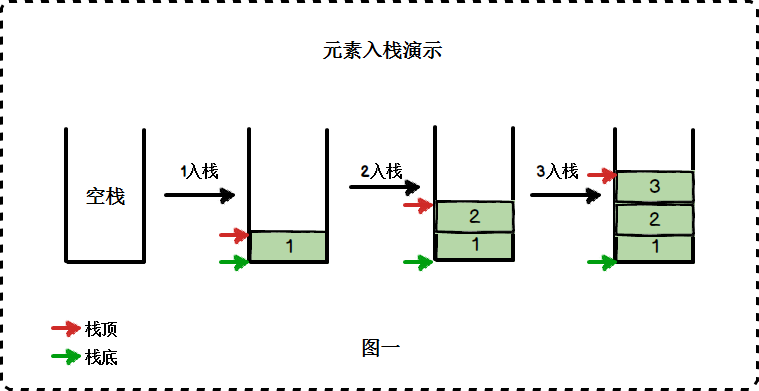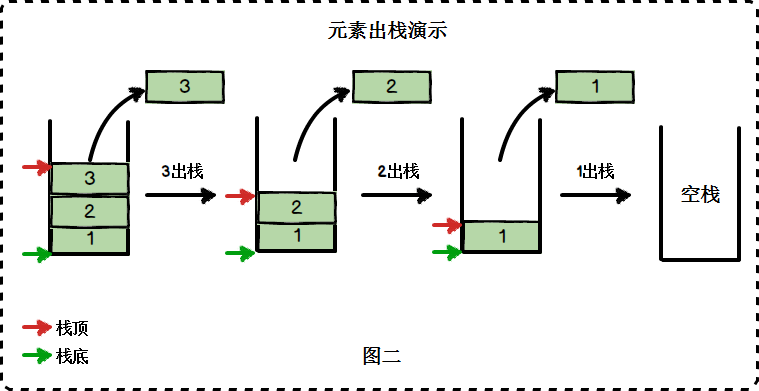# 简介

## 栈的特点

• 栈中的数据元素遵守”先进后出” (First In Last Out) 的原则，简称FILO结构;
• 只能在栈顶进行插入和删除操作。

## 基本操作

• 入栈：在入栈的过程中，栈顶的位置一直在”向上“移动，而栈底是固定不变的。
• 出栈：出栈的顺序为3, 2, 1 ，顺序与入栈时相反，这就是所谓的”先进后出”。 在出栈的过程中，栈顶位置一直在”向下“移动，而栈底一直保持不变。

# 具体实现

• 定义：`stack<int> s;`
• 入栈：`s.push(3);`
• 出栈：`s.pop();`
• 判空：`s.empty();`
• 取大小：`s.size();`

## 系统类型：

``````#include <iostream>
#include <stack>
using namespace std;

int main()
{
stack<int> s;
s.push(1);
s.push(5);
s.push(7);

cout << s.size() << endl;
while(!s.empty())
{
cout << s.top() << " ";
s.pop();
}

return 0;
}
``````

``````3
7 5 1
``````

## 自定义类型：

``````#include <iostream>
#include <stack>
using namespace std;

struct node
{
int val;
node(int _v): val(_v) {}
};

int main()
{
stack<node> s;
s.push(node(1));
s.push(node(5));
s.push(node(7));

cout << s.size() << endl;

while(!s.empty())
{
cout << (s.top()).val << " ";
s.pop();
}

return 0;
}
``````